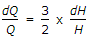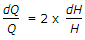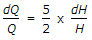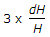# Mechanical Engineering - Hydraulics and Fluid Mechanics

### Exercise :: Hydraulics and Fluid Mechanics - Section 7

26.

The error in discharge (dQ/Q) to the error in measurement of head (dH/H) over a triangular notch is given by

 A.B.C.D.Answer: Option C

Explanation:

No answer description available for this question. Let us discuss.

27.

The separation of flow occurs when the hydrodynamic boundary layer thickness is reduced to zero.

 A. Agree B. Disagree

Answer: Option A

Explanation:

No answer description available for this question. Let us discuss.

28.

If the depth of water in an open channel is less than the critical depth, the flow is called

 A. critical flow B. turbulent flow C. tranquil flow D. torrential flow

Answer: Option D

Explanation:

No answer description available for this question. Let us discuss.

29.

A nozzle placed at the end of a water pipe line discharges water at a

 A. low pressure B. high pressure C. low velocity D. high velocity

Answer: Option D

Explanation:

No answer description available for this question. Let us discuss.

30.

When a body is immersed wholly or partially in a liquid, it is lifted up by a force equal to the weight of liquid displaced by the body. This statement is called

 A. Pascal's law B. Archimede's principle C. principle of floatation D. Bernoulli's theorem

Answer: Option B

Explanation:

No answer description available for this question. Let us discuss.

#### Current Affairs 2021

Interview Questions and Answers